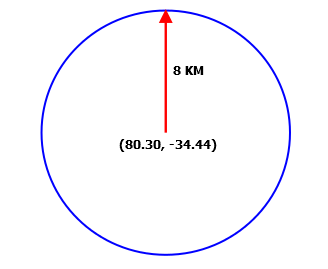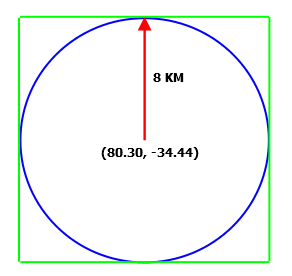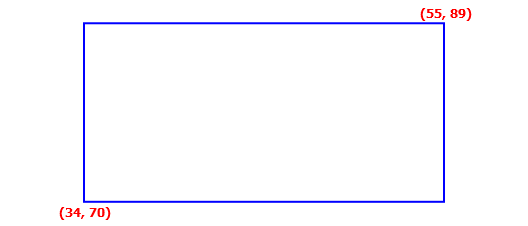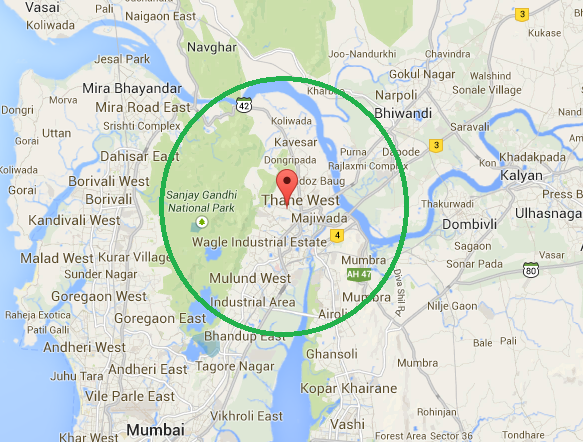# Radius faceting for location based data

Solr provides us the capability to return the faceted search results of the locations that fall within a specific radius from a geographic point, falls in a square with specific dimension, and falls under a rectangular area with specific dimension. In order to understand the concept better, let us learn about the filters and function queries associated based on the scenario.

## Spatial Filters

Following are the parameters that are used when dealing with spatial search:

• d – It holds the radial distance value (in Kilometres)
• pt – It holds the centre point coordinates in latitude-longitude format
• sfield – It holds the spatial indexed field values

### geofilt filter

The geofilt filter allows us to retrieve search results based on geographic distance (that is, radial distance in a circular fashion) from a given point. For instance, if we want to retrieve locations that fall within 8KM from a given point (80.30, -34.44), our query would look something like the following one:

&q=*:*&fq={!geofilt sfield=latlong}&pt=80.30,-34.44&d=8

The outcome of the preceding query can be pictorially represented as shown in the following screenshot:### bbox filter

The bbox filter is quite similar to geofilt, except that it considers the outer square box of the circle to retrieve the associated search information. Let us consider the same scenario was discussed in geofilt in this section. In this case, our example query would look like the following one:

&q=*:*&fq={!bbox sfield=latlong}&pt=80.30,-34.44&d=8

And can be pictorially represented as the following figure:In scenarios wherein we are looking to welcome the search results that fall outside the circle, but within the square box, bbox filter is considered as an alternative of geofilt filter. Using bbox filter, we get an ease in terms of calculating (computing on square is quite easy as compared to a circle).

## Rectangle Filter

You might come across a requirement wherein you need to retrieve the search results that fall within a rectangle formed by two diagonally opposite geographic points. In this scenario, rectangle filter can be used. Let us refer the following diagram that represents our scenario:The preceding diagram or the scenario can be achieved by using the following query:

&q=*:*&fq=latlong:[34,70 TO 55,89]

As an outcome, we retrieve all the locations that fall within the rectangular box.

## Distance function queries

There are three function queries that support spatial search, and are:

• dist – It is used to determine the distance between two geographical points on a plain surface.
• hsin – It is used to compute the distance between two geographical points on a spherical surface.
• sqedist – It is used to compute the square Euclidean distance between two points.

If you wish to know more about Euclidean distance and function queries, we recommend you to refer their Wiki pages at http://en.wikipedia.org/wiki/Euclidean_distance and http://wiki.apache.org/solr/FunctionQuery respectively.

### geodist

geodist is a spatial function is basically used to sort the results based on distance or score and takes three parameters which are optional in nature. These parameters are sfield, latitude, and longitude.

For instance, in order to sort the search results in ascending order of the distance from the given geographical point, we use the following query:

&q=*:*&fq={!geofilt}&sfield=latlong&pt=34,70&d=8

In preceding query chunk will result in all the locations that fall within 8KM radius from the point (34,70) and are sorted such that the nearest location from the given point is rendered on the top and heads till the last location is farthest from the specified point.

By now, we know how to filter our search results based on the radial distance from a given location. This concept will help us understand radius faceting and its implementation.

Let us think of a situation wherein we wish to retrieve faceted information in terms of resident count of the localities that fall under a locality (Thane west). We assume that Thane west holds the geographical coordinates as 19.2189602,72.9681808 in latitude-longitude format. Here in this example, our intention is to retrieve the faceted result that list down the facet values (that is, locality lying within 8KM radius from Thane west) along with the resident count associated to each locality. Let us refer the following diagram that would probably help us understand the scenario better:To achieve our purpose, we append the following query chunk to our base query:

fq={!geofilt sfield=latlong pt=19.221949,72.958276 d=8}&facet=true&facet.field=locality&

The response to the complete query would result in something like the following:

"facet_fields":{
"locality":[
"kavesar",98673,
"purna", 87054,